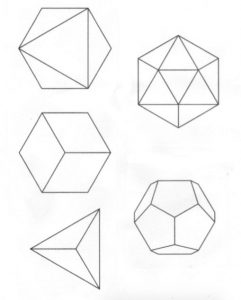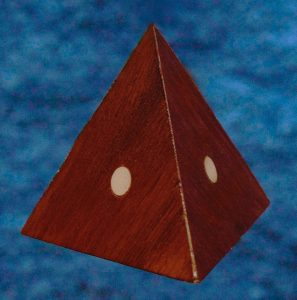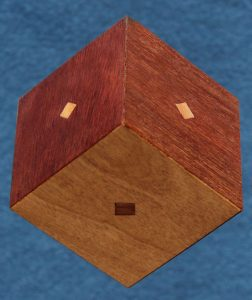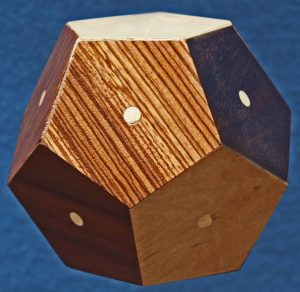The Greek philosopher, Plato, was also a mathematician and he discovered and proved that there are only five regular solids. A regular solid is one that is made up of all the sides being made of one simple regular plane figure such as an equilateral triangle, a square or a regular pentagon. The five regular solids are:

1. Tetrahedron made up of four equilateral triangular sides,
2. Cube (or Hexahedron) made up of six square sides,
3. Octahedron made up of eight equilateral triangular sides,
4. Dodecahedron made up of twelve regular pentagonal sides,
5. Icosahedron made up of twenty regular triangular sides.Here is a picture of all five of them

These shapes have fascinated me for a long time and I decided that it would be an interesting project to make a set of these using different exotic woods for each face. The project required having to design and make two different fixtures to assure that every face was exactly the same size and that the side angles were also exactly the same. (The cube didn’t require any special fixture. I just used my normal table saw settings for that.)

Here are pictures of my five Platonic solids made from different woods. (Each face is about 1/4″ thick.) As an added “secret” touch, I added small beads into each piece before adding the final face. Each piece has the same number of beads as it has faces, so for example: the cube has six beads and the tetrahedron has four beads.TetrahedronCubeOctahedronIcosahedronDodecahedron